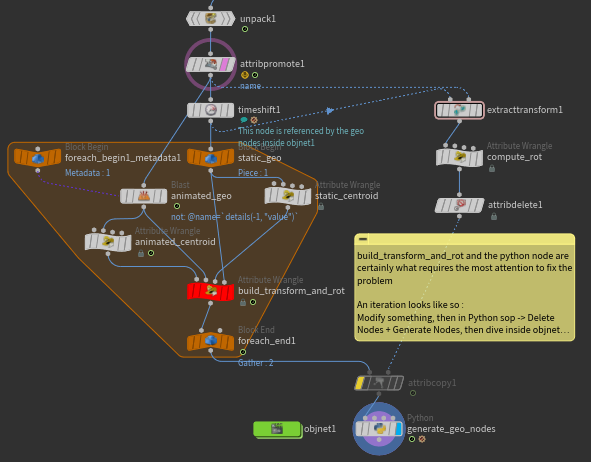Recommended Posts

Hey folks !

I have some animated geometry (in the example scene it's from dop, but any animated geo could be used), and I'm trying to separate the pieces in a subnet of geo nodes, then animate the geo node from the pieces' movement. In other words - extract sop animation to obj level.

I have the geo nodes with the position keyed and working.
It's for the rotation that I'm struggling.

I tried two methods :

1 - I used the extractTransform node, and it's almost working, but there's a slight rotation offset. Why is that so ?

2 - I tried to create the matrix myself and extract the rotation from that using VEX.The matrix building and rotation computing

//Inputs :
// 0 - Animated center
// 1 - Animated geo
// 2 - Static center
// 3 - Static geo
// m = moving | s = static
vector p0m = point(0, "P", 0); // Center (animated)
vector p1m = point(1, "P", 0); // Z axis (animated)
vector p2m = point(1, "P", 1); // Y axis (animated)

vector p0s = point(2, "P", 0); // Center (static)
vector p1s = point(3, "P", 0); // Z axis (static)
vector p2s = point(3, "P", 1); // Y axis (static)

vector zAxism = normalize(p0m - p1m);
vector tempYAxism = normalize(p2m - p1m);
vector yAxism = normalize(cross(tempYAxism, zAxism));
matrix mm = maketransform(zAxism, yAxism, p0m);

vector zAxiss = normalize(p0s - p1s);
vector tempYAxiss = normalize(p2s - p1s);
vector yAxiss = normalize(cross(tempYAxiss, zAxiss));
matrix ms = maketransform(zAxiss, yAxiss, p0s);

//mm -= ms;

vector theRotm = cracktransform(0, 0, 1, p0m, mm);
vector theRots = cracktransform(0, 0, 1, p0s, ms);

theRotm -= theRots;
setpointattrib(0, "rot", 0, theRotm);

But the rotation is not right, it looked like the axis were not the right one (like X belonged to Y or smth). I played around with the vectors order, to no avail.

I'm interested to know why the extractTransform doesn't work correctly, but what I'm really looking forward is to know what's wrong with the second method.

Thanks in advance !

Here's the scene :
extractRot_tests.hip

Create an account or sign in to comment

You need to be a member in order to leave a comment

Create an account

Sign up for a new account in our community. It's easy!

Register a new account